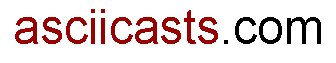#ASCIIcasts

## 15: Fun With Find Conditions

(view original Railscast)

This episode discusses `find` conditions. Below are some lines from `script/console`, shown with the SQL that they generate.

`Task.find(:all, :conditions => ["completed = ? AND priority = ?", false, 2])` `SELECT * FROM "tasks" WHERE (completed = 'f' AND priority = 2)`

Finding all uncompleted tasks with a priority of 2.

The example above works, but what if we’re looking for the `Tasks` that have a `priority` that is `nil`? `Task.find(:all, :conditions => ["completed = ? AND priority = ?", false, nil])` `SELECT * FROM "tasks" WHERE (completed = 'f' AND priority = NULL) `

Searching for null values generates incorrect SQL.

The SQL generated above is incorrect. When searching for null values the correct syntax would be `priority IS NULL`, not `priority = NULL`. Similarly if we were searching for more than one priority value using an array or range we’d have to change the find string to use `IN` rather than `=`, and put brackets around the second question mark in order to ensure that the SQL generated had the correct syntax.

`Task.find(:all, :conditions => ["completed = ? AND priority IN (?)", false, [1,3]])` `SELECT * FROM "tasks" WHERE (completed = 'f' AND priority IN (1,3)`

Since Rails 1.2 there has been a better way to pass conditions: via a hash. Using a hash of conditions ensures that Rails uses the correct conditions when querying the database.

`Task.find(:all, :conditions => { :completed => false, priority => [1,3] }` `SELECT * FROM "tasks" WHERE (completed = 'f' AND priority IN (1,3)`

Using a hash of conditions with an array parameter.

`Task.find(:all, :conditions => {:completed => false, :priority => 2..4})``SELECT * FROM "tasks" WHERE ("tasks"."priority" BETWEEN 2 AND 4 AND "tasks"."completed" = 'f') `

Pass a range and `BETWEEN` will be used in the SQL.

### Using with dynamic finds

Back in episode 2 we used dynamic `find_by` methods. These can also take nil, array or range arguments.

`Task.find_by_priority(1..5)``SELECT * FROM "tasks" WHERE ("tasks"."priority" BETWEEN 1 AND 5) LIMIT 1`

Using a hash of find conditions ensures that Rails generates the correct SQL when passing any type of parameter to the find.